# Discussion of Optimal Monetary Policy under Uncertainty in

• Slides: 13
Download presentation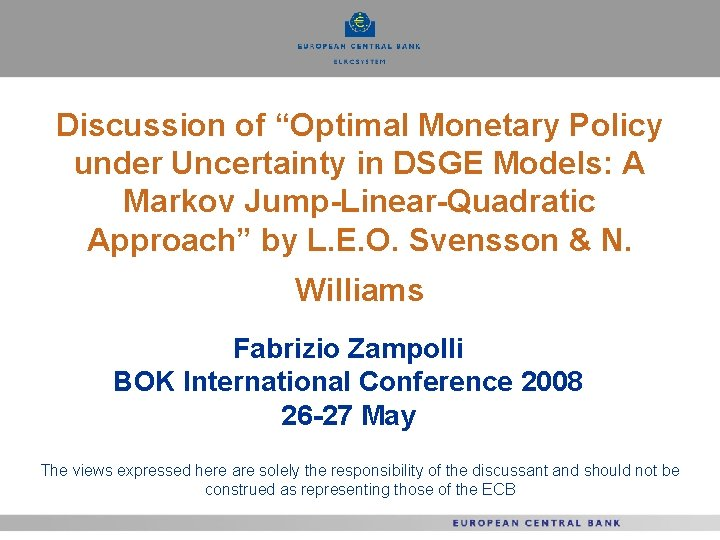Discussion of “Optimal Monetary Policy under Uncertainty in DSGE Models: A Markov Jump-Linear-Quadratic Approach” by L. E. O. Svensson & N. Williams Fabrizio Zampolli BOK International Conference 2008 26 -27 May The views expressed here are solely the responsibility of the discussant and should not be construed as representing those of the ECB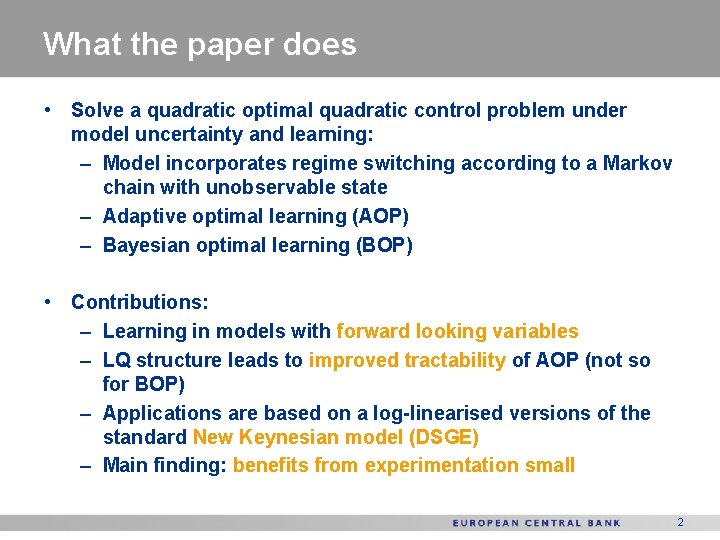What the paper does • Solve a quadratic optimal quadratic control problem under model uncertainty and learning: – Model incorporates regime switching according to a Markov chain with unobservable state – Adaptive optimal learning (AOP) – Bayesian optimal learning (BOP) • Contributions: – Learning in models with forward looking variables – LQ structure leads to improved tractability of AOP (not so for BOP) – Applications are based on a log-linearised versions of the standard New Keynesian model (DSGE) – Main finding: benefits from experimentation small 2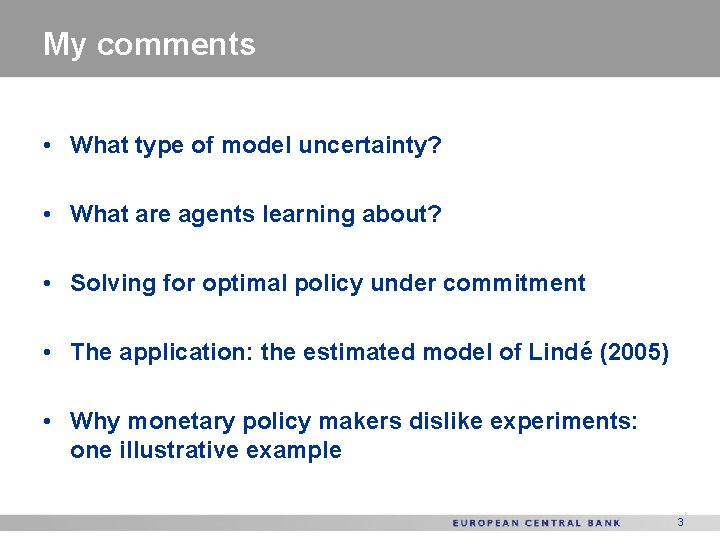My comments • What type of model uncertainty? • What are agents learning about? • Solving for optimal policy under commitment • The application: the estimated model of Lindé (2005) • Why monetary policy makers dislike experiments: one illustrative example 3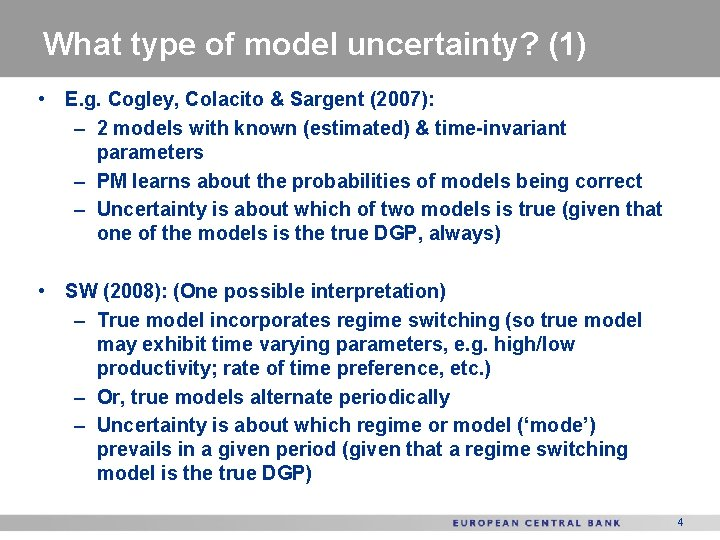What type of model uncertainty? (1) • E. g. Cogley, Colacito & Sargent (2007): – 2 models with known (estimated) & time-invariant parameters – PM learns about the probabilities of models being correct – Uncertainty is about which of two models is true (given that one of the models is the true DGP, always) • SW (2008): (One possible interpretation) – True model incorporates regime switching (so true model may exhibit time varying parameters, e. g. high/low productivity; rate of time preference, etc. ) – Or, true models alternate periodically – Uncertainty is about which regime or model (‘mode’) prevails in a given period (given that a regime switching model is the true DGP) 4What type of model uncertainty? (2) – If true model incorporates regime switching: • Are agents learning within the original non-linearised DSGE model? How? • Main issue: Is a log-linearised regime switching version of such a model a “good” approximation? • Does the approximation capture learning within the model? – Even if shocks are small and modes persistent, not clear that the transition from mode to mode is well approximated • How can we assess the approximation? – [e. g. (perhaps) measure of fit of RS log-linearised model to an artificial sample of data generated by the (calibrated) original RS DSGE model? ? ] 5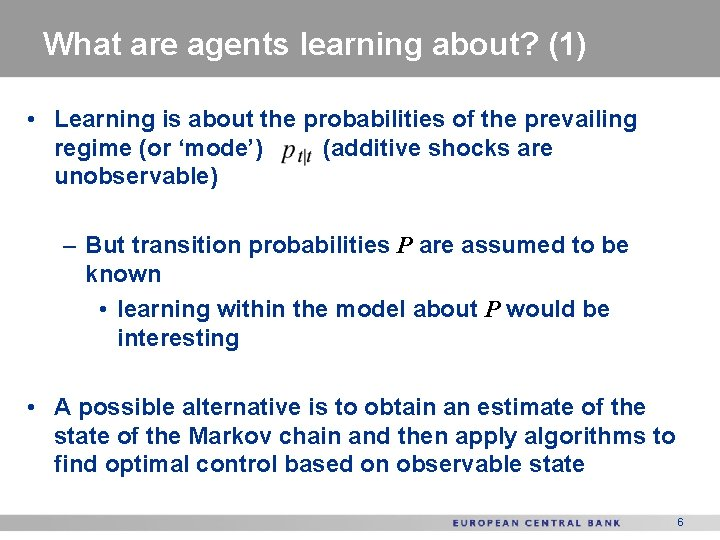What are agents learning about? (1) • Learning is about the probabilities of the prevailing regime (or ‘mode’) (additive shocks are unobservable) – But transition probabilities P are assumed to be known • learning within the model about P would be interesting • A possible alternative is to obtain an estimate of the state of the Markov chain and then apply algorithms to find optimal control based on observable state 6What are agents learning about? (2) For example, get state estimate by solving: Control law depends on an estimate of the state rather than a vector of probabilities Perhaps not much difference with SW approach, yet may make exposition and extensions somewhat simpler? 7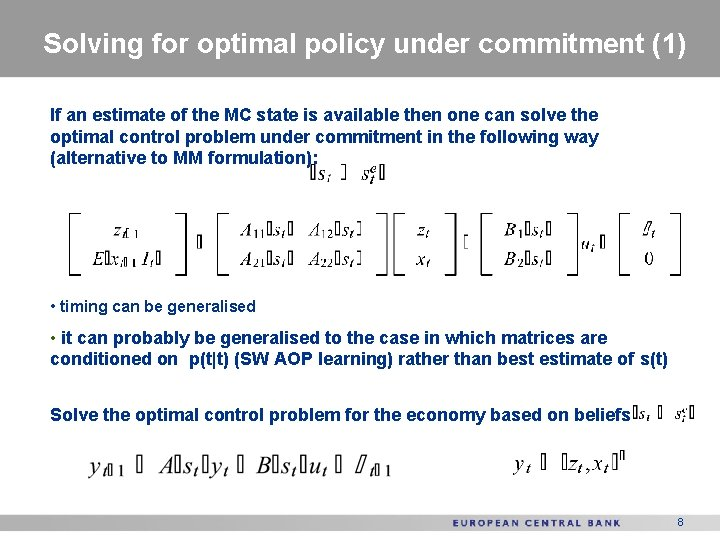Solving for optimal policy under commitment (1) If an estimate of the MC state is available then one can solve the optimal control problem under commitment in the following way (alternative to MM formulation): • timing can be generalised • it can probably be generalised to the case in which matrices are conditioned on p(t|t) (SW AOP learning) rather than best estimate of s(t) Solve the optimal control problem for the economy based on beliefs 8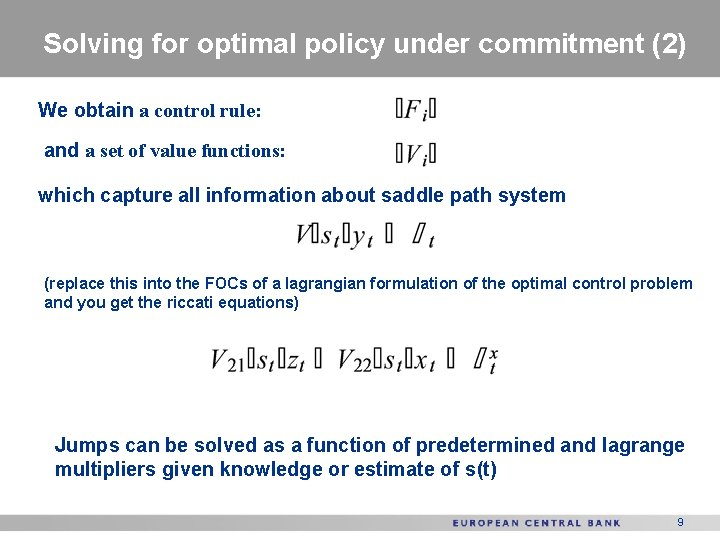Solving for optimal policy under commitment (2) We obtain a control rule: and a set of value functions: which capture all information about saddle path system (replace this into the FOCs of a lagrangian formulation of the optimal control problem and you get the riccati equations) Jumps can be solved as a function of predetermined and lagrange multipliers given knowledge or estimate of s(t) 9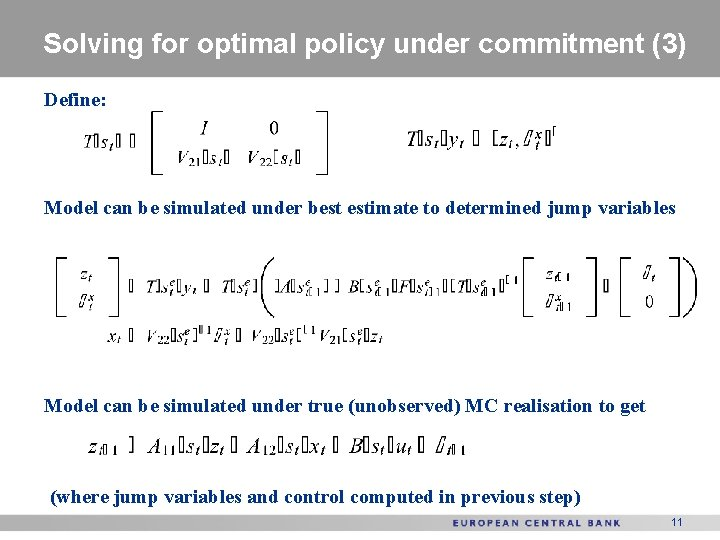Solving for optimal policy under commitment (3) Define: Model can be simulated under best estimate to determined jump variables Model can be simulated under true (unobserved) MC realisation to get (where jump variables and control computed in previous step) 11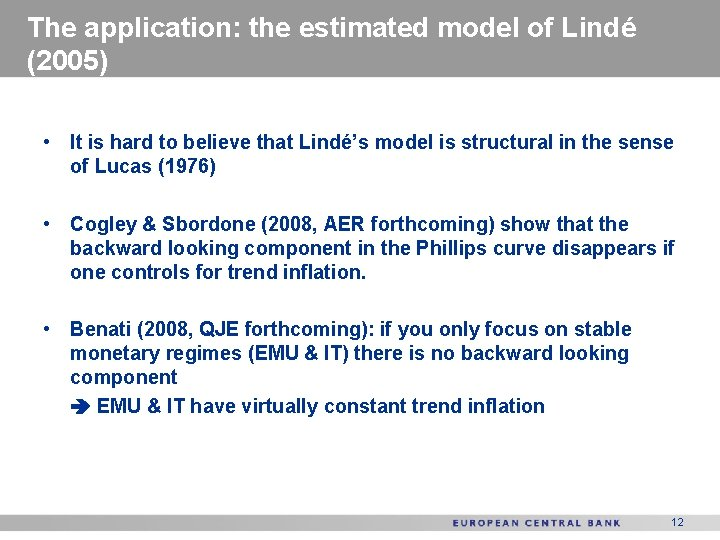The application: the estimated model of Lindé (2005) • It is hard to believe that Lindé’s model is structural in the sense of Lucas (1976) • Cogley & Sbordone (2008, AER forthcoming) show that the backward looking component in the Phillips curve disappears if one controls for trend inflation. • Benati (2008, QJE forthcoming): if you only focus on stable monetary regimes (EMU & IT) there is no backward looking component EMU & IT have virtually constant trend inflation 12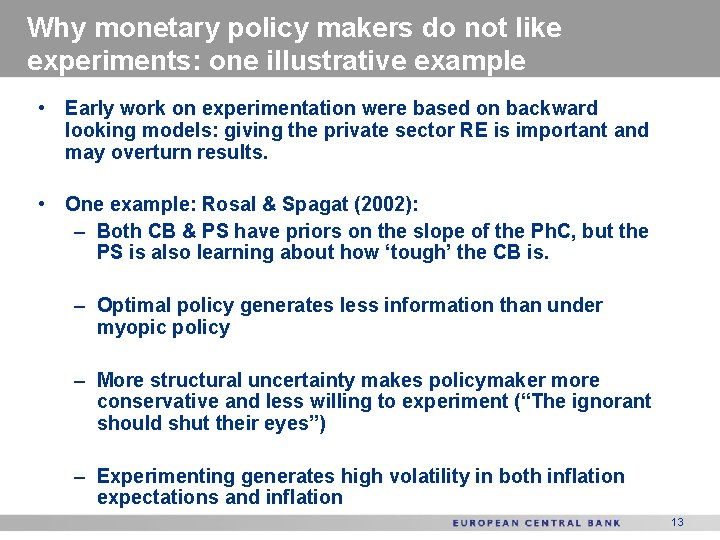Why monetary policy makers do not like experiments: one illustrative example • Early work on experimentation were based on backward looking models: giving the private sector RE is important and may overturn results. • One example: Rosal & Spagat (2002): – Both CB & PS have priors on the slope of the Ph. C, but the PS is also learning about how ‘tough’ the CB is. – Optimal policy generates less information than under myopic policy – More structural uncertainty makes policymaker more conservative and less willing to experiment (“The ignorant should shut their eyes”) – Experimenting generates high volatility in both inflation expectations and inflation 13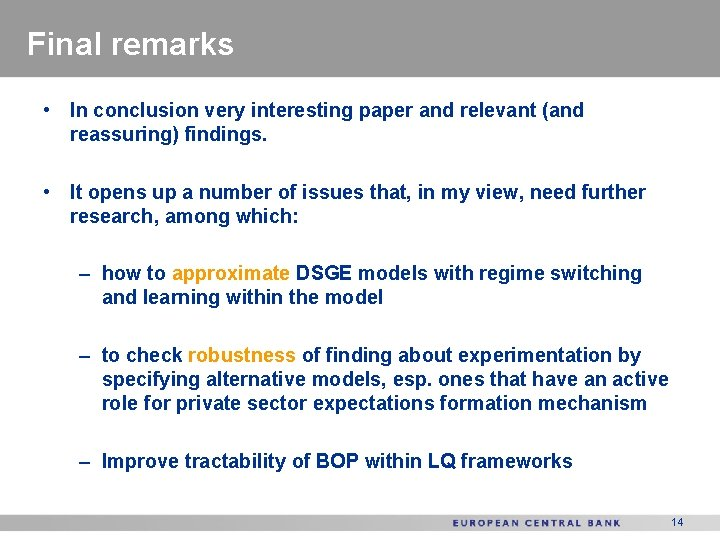Final remarks • In conclusion very interesting paper and relevant (and reassuring) findings. • It opens up a number of issues that, in my view, need further research, among which: – how to approximate DSGE models with regime switching and learning within the model – to check robustness of finding about experimentation by specifying alternative models, esp. ones that have an active role for private sector expectations formation mechanism – Improve tractability of BOP within LQ frameworks 14